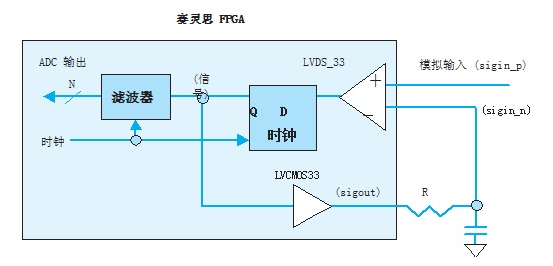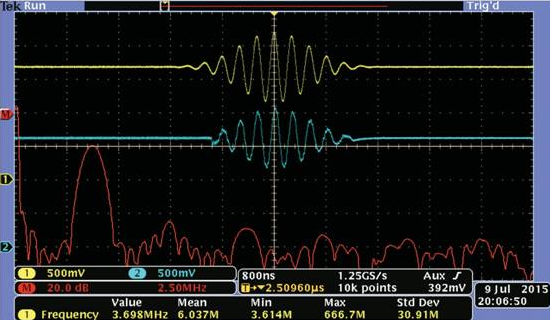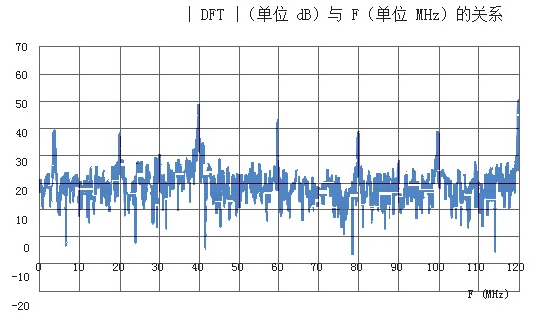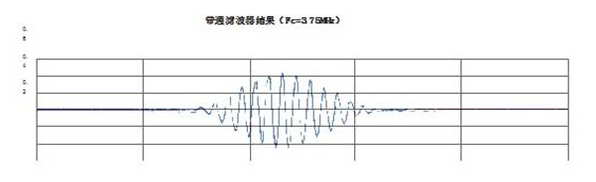# How to digitize hundreds of signals with a single Xilinx FPGA

Using Low Voltage Differential Signaling (LVDS) on new Xilinx FPGAs, the input signal can be digitized with just one resistor and one capacitor. Since hundreds of LVDS inputs are currently available on this generation of Xilinx devices, it is theoretically possible to digitize hundreds of analog signals using a single FPGA.

Our team recently explored a glimpse of possible design areas, digitizing a limited bandwidth input signal with a center frequency of 3.75MHz and a precision of 5 bits, and also studied a variety of output signals for a 128-element linear ultrasound array transducer. Digital solution. Let us first introduce the demo project in detail.

In 2009, Xilinx introduced the LogiCORETM soft IP core. Combined with an external comparator, a resistor and a capacitor, an analog-to-digital converter (ADC) capable of digitizing an input signal up to 1.205 kHz  can be implemented.

Using an FPGA's LVDS input instead of an external comparator, combined with an incremental modulator ADC architecture, uses only one resistor and one capacitor to digitize a much higher frequency analog input signal.

A block diagram of the single-channel delta modulator ADC implemented using the LVDS input on the Xilinx FPGA is shown in Figure 1. Here the analog input drives the LVDS_33 buffer non-inverting input, and the input signal range is essentially 0-3.3V. This method is attractive because it samples the LVDS_33 buffer output at a much higher clock frequency than the analog input signal and outputs it easily through an LVCMOS33 with a small number of components. And because the LVDS_33 input buffer has a relatively high input impedance, in many applications the sensor output can be directly connected to the FPGA input, eliminating the need for a preamplifier or buffer.

The buffer and an external first-order RC filter are fed back to the inverting input of the LVDS_33 buffer. With this circuit, the feedback signal follows the input analog signal by selecting the appropriate clock frequency (F), resistance (R), and capacitance (C).

As an example, Figure 2 shows the input signal (yellow, channel 1) and the feedback signal (blue, channel 2) at F=240MHz, R=2K, and C=47pF. The input signal shown is generated using the Agilent 33250A function generator with its 200MHz, 12-bit arbitrary output function. The Fourier transform of the input signal is calculated by the Tektronix DPO 3054 oscilloscope used by the team and displayed in red (channel M). At these frequencies, the input capacitance (and grounding problem) of the oscilloscope probe does not degrade the quality of the feedback signal displayed by the oscilloscope, but Figure 2 does illustrate the operation of the circuit.

By applying a Blackman-Nuttall window to a 1 Vpp 3.75 MHz sine wave, we define the finite bandwidth input signal shown in Figure 2. Although the noise floor of the windowed signal is theoretically 100 dB less than the amplitude of the center frequency, the sampling frequency and 12-bit accuracy of the Agilent 33250A function generator make the quality of the demo signal far below the theoretical level. Due to the mechanical nature of the transducer, many ultrasonic transducers produce an output signal with a center frequency close to 3.75 MHz which is naturally a finite bandwidth signal and is therefore an ideal source for this approach.Figure 1 - Single Channel Delta Modulator ADC with an external resistor and an external capacitor.Figure 2 - The oscillogram shows the 3.75 MHz input signal (yellow, channel 1) and feedback signal (blue, channel 2) generated by the Agilent 33250A function generator at F=240MHz, R=2K, and C=47pF . The input signal Fourier transform calculated by the Tektronix DPO 3054 oscilloscope is displayed in red (channel M).

We used the Digilent Cmod S6 development module  with the Xilinx SpartanÂ®-6 XC6SLX4 FPGA mounted on a small PCB and used 8 RC networks and input connectors to allow the circular system to digitize up to 8 signals simultaneously , that is, the graph shown in Figure 2 is obtained. Each channel is terminated in parallel with a 50Î© grounding resistor to properly terminate the signal generator's coaxial cable. Note that to achieve this performance, our team set the drive strength of the LVCMOS33 buffer to 24mA and the slew rate to FAST, as documented in the example VHDL source code in Figure 5.

The custom prototype board also supports the use of the FTDI FT2232H USB 2.0 micromodule  for the transmission of packetized serial bit streams to the host PC for analysis. Figure 3 shows the Fourier transform amplitude of the bitstream produced by the prototype board when fed to the analog signal of Figure 2. The peaks associated with the subharmonics of the 240MHz sampling frequency are clearly visible, as well as the peak at 3.75MHz associated with the input signal.Figure 3 - This figure shows the Fourier transform of the bitstream generated by the configuration associated with Figure 2.

a lot of taps

By applying a bandpass finite impulse response (FIR) filter to the bitstream, an N-bit binary representation of the analog input signal can be generated: the ADC output. But since the frequency of the digital bit stream is much higher than the analog input signal, the user needs to use an FIR filter with a large number of taps. However, since the filtered data has only two values, 0 and 1, there is no need to use a multiplier (only the adder is required to add the FIR filter coefficients).Figure 4 - ADC output generated using a 801-tap bandpass filter with a center frequency of 3.75MHz.

The ADC output shown in Figure 4 is generated on the host PC using our 801 tap-band bandpass filter with a center frequency of 3.75MHz designed with the free online FIR filter design tool TFilter. The filter has an attenuation rate of 36dB or higher outside the 2.5MHz - 5MHz passband and a ripple of 0.58dB between 3MHz and 4.5MHz.

The accuracy of the ADC output signal shown in Figure 4 is approximately 5 bits. This is a function of the final oversampling rate, and the user can use a design optimized for lower input frequencies to achieve higher accuracy.

The ADC output signal shown in Figure 4 is also heavily oversampled at 240MHz, which can significantly reduce the ADC output bandwidth. In the hardware implementation of the bandpass filter and the decimation module, the 16th filter can be calculated only when the effective sampling rate is reduced to 1/16 to 15 MHz by decimation (3 times faster than the highest frequency of the finite-band input signal) Output values â€‹â€‹to reduce hardware requirements.

Figure 5 shows the VHDL source code used in conjunction with the Digilent Cmod S6 development module to generate the feedback signal shown in Figure 2 and the bitstream data associated with the Fourier transform of Figure 3. Instantiation of an LVDS_33 input buffer directly

They are connected to the analog input and feedback signals sigin_p and sigin_n respectively. The internal signal sig is driven by the output of the LVDS_33 buffer and is sampled by a built-in flip-flop to generate sigout. The signal sigout is a serial bit stream that is filtered to produce an N-bit ADC output. We implemented the project using the free Xilinx ISEÂ® Webpack tool .

VHDL source code

LIBRARY IEEE ;

USE IEEE.STD_LOGIC_1164.ALL ; LIBRARY UNISIM ;

USE UNISIM.VCOMPONENTS.ALL ;

ENTITY deltasigma IS

PORT (clk :IN STD_LOGIC ;

Sigin_p : IN STD_LOGIC ;

Sigin_n :IN STD_LOGIC ;

Sigout :OUT STD_LOGIC) ; END deltasigma ;

ARCHITECTURE XCellExample OF deltasigma IS SIGNAL sig :STD_LOGIC ;

BEGIN

Myibufds: IBUFDS

GENERIC MAP (DIFF_TERM = "FALSE,

IBUF_LOW_PWR = "FALSE, IOSTANDARD = " "DEFAULT")

PORT MAP (O = " sig,

I = " sigin_p, IB = " sigin_n);

Mydeltasigma:PROCESS(clk) BEGIN

IF (clk = '1' AND clk'EVENT) THEN

Sigout "= sig ; END IF ;

END PROCESS mydeltasigma ; END XCellExample ;

UCF file

NET â€œclkâ€ LOC = J1 |IOSTANDARD = LVCMOS33; NET â€œsigin_pâ€ LOC = N12|IOSTANDARD = LVDS_33; NET â€œsigin_nâ€ LOC = P12|IOSTANDARD = LVDS_33;

NET â€œsigoutâ€ LOC = P7 |IOSTANDARD = LVCMOS33| SLEW = FAST|DRIVE = 24;

Figure 5 shows the VHDL code and the UCF file portion associated with the circuit of Figure 1.

Reduce component count

The ADC architecture described in this paper has been inaccurately cited as a delta-sigma (Î”Î£) type architecture . Although true Î”Î£ ADCs have advantages, the simplicity of this method and the low number of components make it attractive for some applications. And because the LVDS_33 input buffer has a relatively high input impedance, in many applications the sensor output can be directly connected to the FPGA input without the need for a preamplifier or buffer. This is a clear advantage in many systems.

Another advantage of the method in this paper is that the superposition can "mix" multiple serial bit streams, and the output signal can be recovered using a single filter. For example, in an array-based ultrasound system, the serial bit stream can delay the time to implement the focusing algorithm and then add it in a vector fashion so that a digitally focused and focused ultrasound vector can be recovered using a single filter.

Using the FIR filter to generate the ADC output is a simple and intuitive method of violence, which is mainly used for demonstration purposes. In most designs, the ADC output will be generated using a conventional integrator/low pass filter demodulator topology .

Reference material

1. XPS Sigma-Delta (Î”Î£) Analog-to-Digital Converter (ADC) V1.01A, DS587, December 2, 2009

2. R. Steele, Incremental Modulation System, Pentech Press (London), 1975

3. Digilent Cmod S6 Reference Manual, Digilent Inc, September 4, 2014

4. FT2232H Micro Module Product Specification, V1.7, Future Technology Devices InternaTIonal Ltd., 2012

5. TFilter, the free online FIR filter design tool, http://t-filter.engineerjs.com/

6. USE In-depth coaching, UG695 (V13.1), Xilinx, 2011.

7. M. Bolatkale and LJ. Breems, high speed and large bandwidth sigma-delta (Î”Î£) ADC

Full Cover Screen Protector

Guangzhou Ehang Electronic Co., Ltd. , https://www.ehangmobile.com